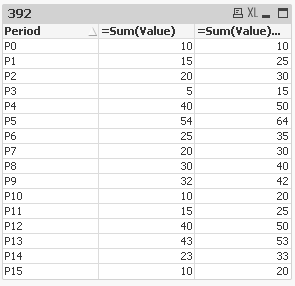# QlikView Scripting

Discussion Board for collaboration on QlikView Scripting.

Announcements

#### Breathe easy -- you now have more time to plan your next steps with Qlik! QlikView 11.2 Extended Support is now valid through December 31, 2020. Click here for more information.Not applicable

## Chart Caluclation

Hi Every one,

I have 5 asset classes in my chart and periods are P0 to P1, out of 5 asset classes 1 is OTC Derivative, when I make selection of OTC and Period=P0 values should be added to other periods(P1 to P15) and you can find current chart and it should be change to my requirement.

Make sure that this change should not reflect to other selections.

Raj

1 Solution

Accepted SolutionsMVP

## Re: Chart Caluclation

Script for reference:

Table:

LOAD * INLINE [

Period, Value

P0, 10

P1, 15

P2, 20

P3, 5

P4, 40

P5, 54

P6, 25

P7, 20

P8, 30

P9, 32

P10, 10

P11, 15

P12, 40

P13, 43

P14, 23

P15, 10

];

Created a straight table with 2 expressions:

1) =Sum(Sales)

2) =Sum(Value) + If(Period = 'P0', 0, RangeSum(Above(Value, RowNo() - 1)))

Second expression will add P0 to everything from P1 to P15 (Snapshot attached)Let me know if this is useful.

Best,

S

36 RepliesMVP

## Re: Chart Caluclation

I am sorry, but I don't really understand what you are trying to achieve here. When you make a selection on the Asset Class = OTC and Period =P0, what are you expecting to happen?

Best,

SNot applicable

## Re: Chart Caluclation

Hi S,

For ex: P0=10, P1=20,P2=5 then if select on OTC P0 value should be added to P1 to other periods.

Like our ex: after selection(OTC) P0=10 , P1=30(P0+P1), P2=15(P0+P2).

Thank you,MVP

## Re: Chart Caluclation

Is this close to what you want? PFA

Best,

SNot applicable

## Re: Chart Caluclation

Hi S,  Not able to open your QVW due to license, could you please help me in another way.   Thank youMVP

## Re: Chart Caluclation

Script for reference:

Table:

LOAD * INLINE [

Period, Value

P0, 10

P1, 15

P2, 20

P3, 5

P4, 40

P5, 54

P6, 25

P7, 20

P8, 30

P9, 32

P10, 10

P11, 15

P12, 40

P13, 43

P14, 23

P15, 10

];

Created a straight table with 2 expressions:

1) =Sum(Sales)

2) =Sum(Value) + If(Period = 'P0', 0, RangeSum(Above(Value, RowNo() - 1)))

Second expression will add P0 to everything from P1 to P15 (Snapshot attached)Let me know if this is useful.

Best,

SNot applicable

## Re: Chart Caluclation

Hi S,

Thanks for the above expression and its meaningful but in my case, I am not able to understand for my below expression.

Thank youMVP

## Re: Chart Caluclation

I assume the value corresponds to one of these based on the selection (Trad_UNREALIZED_PL, Trad_COLLATERAL_VALUE, Trad_CURR_BOOK_VALUE)? If it does then you can do something like this:

If(Trad_PRODUCT_TYPE = 'OTC Derivative',

Sum(Value) + If(Period = 'P0', 0, RangeSum(Above(Trad_UNREALIZED_PL, RowNo() - 1))), If(Trad_PRODUCT_TYPE = 'SFT',

Sum(Value) + If(Period = 'P0', 0, RangeSum(Above(Trad_COLLATERAL_VALUE, RowNo() - 1))),

Sum(Value) + If(Period = 'P0', 0, RangeSum(Above(Trad_CURR_BOOK_VALUE, RowNo() - 1)))))

Is this something you are looking to do? If not then I would suggest that you share some sample data so that the actual requirement can be pin-pointed.

Best,

SNot applicable

## Re: Chart Caluclation

Hi S,

Thanks for the reply.

Data is huge so I can explain with screen-shot.

I have two dimensions 1. Period, 2. Trad_Exclusion_Rule(Dragged to up: 0,100,150...,). When I make selection(OTC) then P0 should be add to other periods(p1+p0,p2+p0...,). If I make selection of other Asset class and it should not affect.

my expression as above.

Your expression is not worked out:

If(Trad_PRODUCT_TYPE = 'OTC Derivative',

Sum(Value) + If(Period = 'P0', 0, RangeSum(Above(Trad_UNREALIZED_PL, RowNo() - 1))), If(Trad_PRODUCT_TYPE = 'SFT',

Sum(Value) + If(Period = 'P0', 0, RangeSum(Above(Trad_COLLATERAL_VALUE, RowNo() - 1))),

Sum(Value) + If(Period = 'P0', 0, RangeSum(Above(Trad_CURR_BOOK_VALUE, RowNo() - 1)))))

Thank youMVP

## Re: Chart Caluclation

If(Trad_PRODUCT_TYPE = 'OTC Derivative',

Sum(Trad_UNREALIZED_PL) + If(Period = 'P0', 0, RangeSum(Above(Trad_UNREALIZED_PL, RowNo() - 1))), If(Trad_PRODUCT_TYPE = 'SFT',